## Towards Certified Digital Audio Processing

### Emilio Jesús Gallego Arias (joint work with Pierre Jouvelot)

#### MINES ParisTech, PSL Research University, France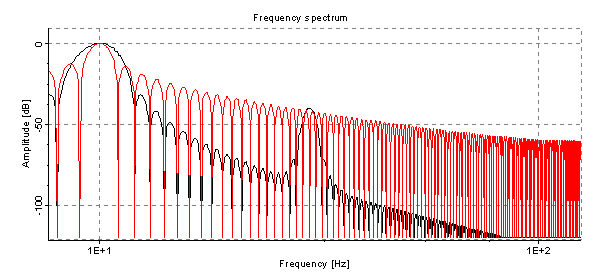##### December 5th 2016 - Synchron 2016 - Bamberg

FEEVER ANR Project

Real Time Signal Processing
$\cap$ Programming Language Theory
$\cap$ Theorem Proving

Key Goal: Arbitrary Formal Proofs

• Linearity:
• A set of programs correspond to an LTI system.
• Simulation:
• Different executions/implementations denote the same program. Including compilation!
• Approximation:
• A program approximates another program or mathematical system. Quite free-form! Think of relating a program to its Z-transform.

## Basic Audio Objects

###### Recursive 2nd order Filter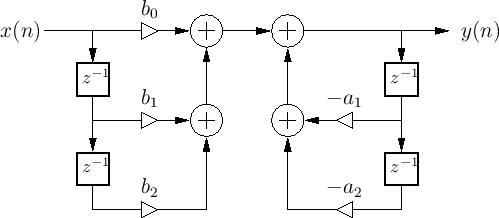Image Credits: Julius Orion Smith III

###### Wave-guide Resonator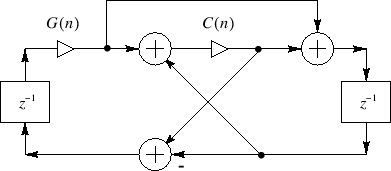Image Credits: Julius Orion Smith III

##### Programs correspond to synchronous data-flow.

Julius Orion Smith III audio-focused book's series:

• Mathematics of the Discrete Fourier Transform (DFT)
• [Partially handled + missing inf. sum.]
• Introduction to Digital Filters
• [Work of this talk]
• Physical Audio Signal Processing
• [Minor bits in this talk + missing ODE]
• Spectral Audio Signal Processing
• [Future work]
##### Main Relevant Points:
• Free mix of mathematics and computation.
• Linear algebra is pervasive.
• Proofs of uneven difficulty, not constructive-friendly.

## Where to Look?

### VeriDrone Project[Ricketts, Machela, Lerner, ...]

[EMSOFT 2016] traces+LTL, continuity, Lyapunov.

### Lustre Certified Compiler[Bourke et al.]

Work in progress, denotational semantics?

### FRP/Arrows[Krishnaswami, Elliot, Hughes, Hudak, Nilsson, ...]

Nice functional PL techniques; too abstract for real-time sample-based DSP.

### Synchronous Languages [Berry, Caspi, Halbwachs, Pouzet, Guatto, ...]

Guatto's PhD Most related to our approach. Suited to linear algebra/DSP?
Data-intensive vs control-intensive require quite different control techniques. [Berry, 2000]

### Isabelle/HOL[Akbarpour, Tahar et al.]

Fixed systems, numerical issues.

## The Plan

##### Formal Proof and Semantics
$$\forall p, ⟦p⟧_A = ⟦T(p)⟧_B$$

Main question, what is ⟦ ⟧?

##### Borrow Techniques from Functional Programming to Attack the Problem:
• Define a functional, dataflow-like language.
• Untyped operational semantics!
• Classify well-behaved programs wrt the enviroment.
• Logical Relations
• ?
• Profit!
Replace stratification by domain theory/types by operational step-indexing.

## What now: Pre-Wagner

Simple λ-calculus with feedback, pre, and stable types.

#### Types and Syntax:

$$\newcommand{\ip}{\langle{#1},{#2}\rangle} \newcommand{\inferrule}{\frac{#1}{#2}} \newcommand{\fpo}{\varphi} \newcommand{\fpw}{\psi} \newcommand{\fpt}{\theta} \newcommand{\vm}{\vec{m}} \newcommand{\vn}{\vec{n}} \newcommand{\vo}{\vec{o}} \newcommand{\vx}{\vec{x}} \newcommand{\ve}{\vec{e}} \newcommand{\vv}{\vec{v}} \newcommand{\rn}{{~\mathsf{#1}}} \newcommand{\fpre}{{#1}_{\{#2\}}} \newcommand{\fjud}{\{ #1 \} ~~ {#2} ~~ \{ #3 \}} \begin{array}{lrll} T & := & R \mid I_n \mid R[n] & \text{Samples, Ordinals, Arrays} \\ & ∣& R@r & \text{rated stream} \\ & ∣& T ⊗ T & \text{product} \\ & ∣& R → T & \text{simple function} \\ & ∣& R@r ⇒_m T & \text{stream processor} \\ \end{array}$$ $$\newcommand{\R}{\mathbf{R}} \newcommand{\feed}{\text{feed}~{#1} = {#2}} \newcommand{\let}{\text{let}~{#1}={#2}~\text{in}~{#3}} \newcommand{\feedin}{\text{feed}~{#1} = {#2}~\text{in}~{#3}} \begin{array}{lrll} e & := & x \mid c \mid p & \text{Var, Const, Prim} \\ & \mid & \pi_n~e \mid (e, e) & \text{Products} \\ & \mid & λ x.~e \mid (e_f~e_a ) & \text{Regular functions} \\ & \mid & Λ x.~e \mid \{e_1, …, e_n\} & \text{Stream functions} \\ & \mid & e_{\{j\}} \mid \feed{\vec{x}}{\vec{e}} & \text{Previous, Feedback} \end{array}$$

## Pre-Wagner: Op. Semantics

Assume causal feedback. Big-step, time-indexed relation. Every program reduces to a value at time step $n$. Similar to synchronous data-flow.

$$\newcommand{\ev}{{#1} \downarrow_{#2} {#3}} \begin{gather} \inferrule { } {\ev{x}{n}{x}} \qquad \inferrule {\ev{e_1}{n}{v_1} \quad … \quad \ev{e_k}{n}{v_k} \quad P(v_1, …, v_1) ↓ v } {\ev{P(e_1,…,e_k)}{n}{v}} \\[1em] \inferrule {\ev{eₐ}{n}{vₐ} \quad \ev{e_f[x/vₐ]}{n}{v}} {\ev{(λ~x.e_f)~eₐ}{n}{v}} \qquad \inferrule {\ev{eₐ}{n}{vₐ} \quad \ev{e_f[x/vₐ]}{n}{v}} {\ev{(Λ~x.e_f)~eₐ}{n}{v}} \\[1.5em] \inferrule { \ev{e_1}{n}{v_1} ~\ldots~ \ev{e_k}{n}{v_k} } { \ev{ \{e_1, \ldots, e_k \} }{n}{\{v_1, \ldots, v_k \} } } \qquad \inferrule { } {\ev{e_{\{k+1\}}}{0}{0_e}} \qquad \inferrule {\ev{e_{\{k\}}}{n}{v}} {\ev{e_{\{k+1\}}}{n+1}{v}} \\[2em] \inferrule {\ev{\ve[\vx/0_\ve]}{0}{\vv}} {\ev{\feed{\vx}{\ve}}{0}{\vv}} \qquad \inferrule { \ev{\feed{\vx}{\ve}}{n}{\vv} \quad \ev{\ve[\vx/\vv]}{n+1}{\vv} } { \ev{\feed{\vx}{\ve}}{n+1}{\vec{v}} } \end{gather}$$

## Examples: DF-I Filter$$\mathsf{df1} ≡ λ~(a:R[k_a])~(b : R[k_b]). Λ x.~\feed{y}{ \{ b \cdot x + a \cdot y} \}$$

Note size polymorphism of the dot operator:

$$x \cdot y ≡ \sum_i^k x[i] ⬝ \fpre{y}{i}$$

## Examples: DF-II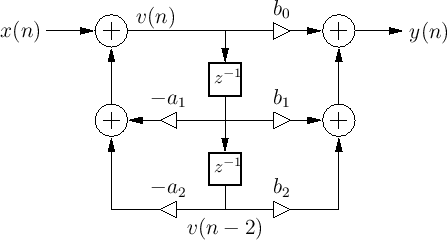$$\mathsf{df2} ≡ Λ x.~ \feedin{v}{ \{ x + a \cdot v \}}{ \{ b \cdot v \} }$$

Compare with df1:

$$\mathsf{df1} ≡ Λ x.~\feed{y}{ \{ b \cdot x + a \cdot y} \}$$

## Examples: Wave-Guide OSC$$\begin{array}{rcl} \text{feed}~x & = & C \cdot (G \cdot x' + y') - y' \\ y & = & C \cdot (G \cdot x' + y') + G \cdot x' \end{array}$$

we write $x' \equiv \fpre{x}{1}, y' \equiv \fpre{y}{1}$. With different sugar:

$$\feed{z}{ \{ (C \cdot (G \cdot z'_1 + z'_2) - z'_2, C \cdot (G \cdot z'_1 + z'_2) + G \cdot z'_1) \} }$$

## Towards a New Semantics Or some critics of "pre"

A) Compare a typical FIR:

$$x \cdot y ≡ \sum_i x[i] ⬝ \fpre{y}{i} \qquad \text{vs} \qquad x \cdot y ≡ \sum_i x[i] ⬝ y[i]$$
• Model where pre behaves like array indexing?

B) Evaluation strategy and caching?

$$\begin{array}{ll} \text{collect} &: R ⊗ \dots ⊗ R ⇒_0 R \\ \text{avg} &: R ⇒_{100} R \\ \text{process} &: R ⊗ \dots ⊗ R ⇒_? R \\ \text{process} &= avg ∘ collect \end{array}$$

What should be the type of $\text{process}$ ? A caching of 100? Ups!

C) How can we drop the causality requirement? "pre" doesn't need it!

## Val-Wagner

Assume CBV, only variables can be time-addressed:

#### Types and Syntax:

$$\begin{array}{lrll} T & := & R \mid I_n \mid R[n] & \text{Samples, Ordinals, Arrays} \\ & ∣& R@r & \text{rated stream} \\ & ∣& T ⊗ T & \text{product} \\ & ∣& R → T & \text{simple function} \\ & ∣& R@r ⇒_m T & \text{stream processor} \\ \end{array}$$ $$\begin{array}{lrll} e & := & \fpre{x}{k} \mid c \mid p & \text{Var, Const, Prim} \\ & \mid & \pi_n~e \mid (e, e) & \text{Products} \\ & \mid & λ x.~e \mid (e_f~e_a ) & \text{Regular functions} \\ & \mid & Λ x.~e \mid \{e_1, …, e_n\} & \text{Stream functions} \\ & \mid & \feed{\vx}{\ve} & \text{Feedback} \end{array}$$

## Where is my Pre?

Variables are arrays with history; we recover "pre" by "eta":

$$\fpre{e}{k} ≡ (Λ x.~\fpre{x}{k})~e$$

Untyped Operational Semantics using a stack of arrays ${\cal E}$:

$$\inferrule{ }{ ⟨{\cal E} ∥ \fpre{x}{k}⟩ ↓_n {\cal E}(x)[k] } \\[1.1em] \inferrule{ ⟨{\cal E} ∥ f ⟩ ↓_n λ x. v_f \quad ⟨{\cal E} ∥ e ⟩ ↓_{(0 ≤ i ≤ n)} {v_e}_i \quad ⟨[{v_e}_0, …, {v_e}_n] ∷ {\cal E} ∥ v_f ⟩ ↓_n v }{ ⟨{\cal E} ∥ f~e ⟩ ↓_n v } \\[1.1em] \inferrule{ ⟨{\cal E} ∥ \feed{\vx}{\ve} ⟩ ↓_{(0 ≤ i ≤ n)} {\vec{v_e}}_i \quad ⟨[{\vec{v_e}}_0, …, {\vec{v_e}}_n, \vec{0}] ∷ {\cal E} ∥ \ve ⟩ ↓_{n+1} \vv }{ ⟨{\cal E} ∥ \feed{\vx}{\ve} ⟩ ↓_{n+1} v }$$

Feedback is interpreted now by "sequential" execution! We allow it to update the environment. We can alternatively replace $ε$ for $\vec{0}$.

## Interaction and Typing

Environment ${\cal E}$ interacts well with $e$ if it contains enough history.

$${\cal E}~ \bot_n~ e ↔ ∃ v, ⟨ {\cal E} ∥ e ⟩ ↓_n v$$

#### Type System:

Co-effects keep track of history-access. Array subtyping and polarity (optional):

$$\begin{gather} \inferrule { k \leq n } { (Γ_\vm, x :_n R@r ⊢ \fpre{x}{k} : R[r] } \rn{Var} \qquad \inferrule { (Γ_\vm, x :_n R@r ⊢ e : T } { Γ_\vm ⊢ Λ x.~e : R@r ⇒_n T } \rn{Sp_I} \qquad \inferrule { \Gamma_\vm, x :_0 R[r(n+1)] ⊢ e : T} { \Gamma_\vm, x :_n R@r ⊢ e : T}\rn{Sub} \end{gather}$$

#### Main Lemma:

If $Γ_\vm ⊢ e : T$ and $Γ_\vm \vdash {\cal E}$ and $e$ is causal (definition of your choice!) then $$∀ n. {\cal E}~ \bot_n~ e \qquad \qquad \qquad (\equiv {\cal E}~ \bot~ e)$$

Semantics for types $⟦T⟧$: sets closed under $⊥$.

## Case Study I: Linearity

We characterize the set of linear Wagner programs, a first step for the Z-transform theory of linear programs.

Lin-Wagner is obtained by dropping constants and non-linear operations. What is the right definition of linearity? We use a logical relation:

$$\begin{array}{lcl} \mathsf{linR_{n}}^{\vec{R}}(e) &≡& \forall {\cal E}_1~{\cal E}_2.~ ⟨{\cal E}_1 ∥ e⟩_n - ⟨{\cal E}_2 ∥ e⟩_n = ⟨{\cal E}_1 - {\cal E}_2 ∥ e⟩_n \\ \mathsf{linR_{n}}^{\vec{R} ⇒ \vec{R}}(f) &≡& \forall {\cal E}_1 ~{\cal E}_2 ~r_1 ~r_2.\\ && \quad ⟨{\cal E}_1 ∥ f~r_1⟩_n - ⟨{\cal E}_2 ∥ f~r_2⟩_n = ⟨{\cal E}_1 - {\cal E}_2 ∥ f~(r_1 - r_2)⟩_n \end{array}$$

The relation is simple, but works at higher-types. Every Lin-Wagner program satisfies the relation! The proof proceeds, first, by induction on the typing derivation, then by induction on the number of steps.

## Case Study I: Linearity

What about multi-linearity? A brief comment:

Compare: $$\begin{array}{l} λ x. Λ y . x \cdot y : R → S ⇒ T \\ Λ x. Λ y . x \cdot y : R ⇒ S ⇒ T \end{array}$$

## Case Study II: The SSSM Abstract Machine

Lin/Val-Wagner semantics compute the full history of the argument at each function call. We introduce a heap and define a monadic semantics for types:

The binary heap follows the structure of expressions.

## Case Study II: The SSSM Abstract Machine

Evaluation as usual. Operations to "focus" the heap, add a new value, etc...

Idea: put/get respect initial buffer size.

## Case Study II: The SSSM Abstract Machine

A well-formed heap for that program contains the proper amount of buffering for $e$, plus the correct values for the past. The main lemma is then:

$${\cal E} ≈_n ({\cal D, H}) → ⟨ {\cal E} ∥ e ⟩_{n+1} = \mathsf{exprV~{\cal D}~e}~H$$

## Case Study III: Frequency Domain

#### Quick Review:

• Frequency Response = impulse response output.
• A filter is stable = its Frequency Response is summable.
• Stability implied by the existence of the DTFT.
• The DFT may approximate the DTFT.
• The DTFT is the evaluation of the Z-transform for the unit circle.

#### Current state:

• Previous linearity theorem and a notion of DFT in Coq are prerequisites.
• Work in progress, Z-semantics defined propositionaly.
• Requires a representation theorem to interpret types.

## Conclusions

• Long path, technique is powerful. Applications to other areas, extensions (references, polymorphism).
• Multirate/clocks: Very simple reclocking primitives, enough for our needs. Better support under study.
• Some other limitations: false cycle circuits.
• Implementation in Ocaml with a core type system. Interesting design space, in particular wrt to closures.
• Coq formalization: working but more experience is needed to decide on some implementation details.
• Connection to State Space analysis, control theory, MIMO systems.
• Floating Point Interpretation. Numerical issues are pervasive in this domain, but trying to get there.
• Verification of program and transform optimization. [Püschel]

## The fun starts !!

We embed Val-Wagner into Coq using a lightweight dependenly typed syntax. We focus on a reduced subset, leaving higher order, rates, and stable values for later. Recall that: $R ⇒ R ⇒ R ≈ R ⊗ R → R$. Thus:

## Program Interpreation:

The lightly-typed syntax allows to overcome typical termination issues in our embedding and provide an "executable" semantics inside Coq, we want something like:

$$⟦ \_ ⟧_E : ∀~\mathsf{τ}~({\cal E} : \mathsf{env})~(\mathsf{e} : \mathsf{expr~τ})~(\mathsf{n} : ℕ), ⟦ \mathsf{τ} ⟧_T$$

We take a simple choice and use $\mathsf{env} = \mathsf{seq}~(\mathsf{seq}~ℝ)$. For type interpretation we use:

$$\begin{array}{lcl} ⟦ ⊗~n ⟧_T &= & \mathsf{cV[ℝ]_n} \\ ⟦ n_a ⇒ n_r ⟧_T &= & \mathsf{seq~cV[ℝ]_{n_a} → cV[ℝ]_{n_r}} \\ \end{array}$$

Thus functions have access to all history. We also provide an initial value for every type. Many more refinements are possible!

## Step-Indexing

The definition of step-indexing in Coq is tricky, interpretation proceeds by induction on time and expressions. We must use a stronger induction principle for Coq to be happy:

## Geometric Signal Theory

#### The DFT:

$$X(\omega_k) = \ip{x^T, \omega_k} ~~\text{where}~~ \omega_k = \omega^{k*0},\ldots,\omega^{k*(N-1)}$$

Most properties are an immediate consequency of matrix multiplication lemmas in math-comp.

##### Primitive roots:

The constructive algebraic numbers in mathcomp provides us with a primitive root of the unity such that $ω^N = 1$.

## In an Ideal World...

#### ... we'd have everything we need in our library.

• We are not so far from it.
• Took the definitions from classfun.v and proved:

Energy theorems are easy corollaries. Compact development for now, see the library.

## Transfer Function:

We now want to relate our programs to their transfer function. The first step is to define the Z-interpreation for types:

We'd like a theorem relating the summability of:

to the evaluation of the transfer function. We can establish:

## Z-Interpretation of Programs

It is work in progress; we tried to build an effective procedure, it is difficult.

## First Try: Mini-Faust [LAC2015]

• Faust [Orlarey 2002]: DSL for DSP arrow-like, point-free combinators.
• Coq semantics: step-indexing, math-comp.

### Problems with the approach:

• DSP not friendly to point-free combinators (matrices).
• PL methods awkward for DSP experts.
• Doesn't work well for complex examples.
• Key point for proofs: Semantics of programs.

## Executing Equations:

This system is simple but convenient due to its natural, computable, and canonical embedding, what can be done in the case of Lustre-like equations?

1. Define a topological type $\mathsf{ts}$ and sort function $\mathsf{topo : eqn \to option~ ts}$, from sets of equations to schedules.
2. Define an interpreter for $\mathsf{ts}$.
3. [Optional]: If the program is well-clocked, then $\mathsf{topo}$ succeeds.
4. Prove a): Prove topo is sound (the output respects the original eqn).
5. Prove b): All possible schedules return the same value in the interpreter. (invariance under permutation)
6. The rest of the proof should be "free" of scheduling choices. The intrepreter should expose the internal state from the begining.

## Handling Clocked Streams

##### [The beginning of it all]

Port of the Lucid paper to Coq/Ssreflect; replace dependently typed streams by sequences plus a decidable well-clocked relation:

Decidability provides "inversion views" (also called small inversion sometimes) which are very convenient.

## Example: Filter Stability

$$\fjud{\fpo}{f}{\fpw} \iff \forall t, \fpo(i(t)) \Rightarrow \fpw(f(i)(t))$$

In PL terms: $\fjud{x \in [a,b]}{\mathsf{*(1-c) : + \sim *(c)}}{x \in [a,b]}$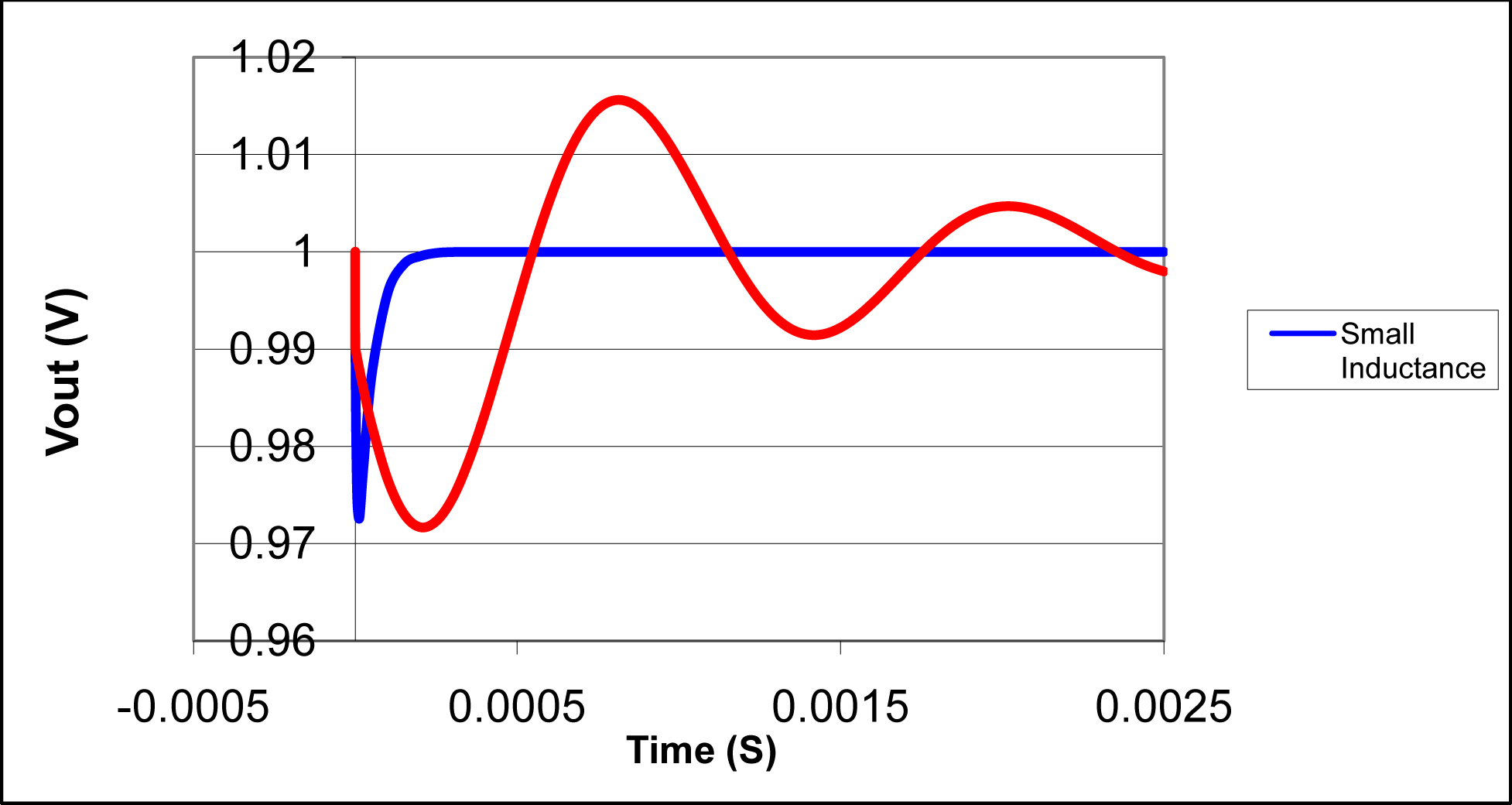In DSP terms: The impulse response decays to 0 as time goes to infinity.

• Higher-order filters may make PL methods impractical.
• State seen as matrices/arrays.

## Context of the ANR project:

Practice of real time DSP still far from convenient.

##### Starting point, Faust:
• Faust [Orlarey 2002], a functional PL for audio programming.
• Abstracts away low-level complexity, efficient execution.
• A success, good number of users, high-interest topic. (CCRMA workshops, Kadenze course, etc...)
##### Faust's Future:
• Extend Faust to multirate processing.
• Reasoning about audio programs is not easy.

/